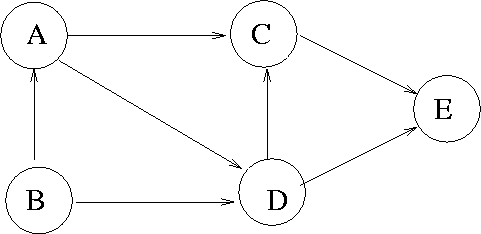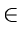Next:7.5.3 Strong ComponentsUp:7.5 Directed Acyclic GraphsPrevious:7.5.1 Test for Acyclicity

## 7.5.2 Topological Sort

Topological sort is a process of assigning a linear ordering to the vertices of a DAG so that if there is an arc from vertex i to vertex j, then i appears before j in the linear ordering
• Useful in scheduling applications
• Example: Consider the DAG in Figure 7.14.
•A topological sort is given by: B, A, D, C, E. There could be several topological sorts for a given DAG

• Topological sort can be easily accomplished by simply including an additional statement in the depth first search procedure of the given graph.
• Let number [vertex] be the number that we assign in topological sort. We use a global integer variable n, whose initial value is zero.

int n = 0;

void topsort (vertex v);

/* assigns numbers to vertices accessible from v in

reverse topological order */

vertex w;

{

mark[v] = visited;

for (wL[v])

if (mark[w] == unvisited)

topsort (w);

number[v] = n+1

}

• This technique works because a DAG has no back arcs.
Consider what happens when the DFS leaves a vertex x for the last time. The only arcs emanating from v are tree, forward, and cross arcs. But all these arcs are directed towards vertices that have been completely visited by the search and therefore precede x in the order being constructed.Next:7.5.3 Strong ComponentsUp:7.5 Directed Acyclic GraphsPrevious:7.5.1 Test for Acyclicity
eEL,CSA_Dept,IISc,Bangalore